Zeroes of a Polynomial and Remainder Theorem - Polynomials, Class 9, Mathematics

# Zeroes of a Polynomial and Remainder Theorem - Polynomials, Class 9, Mathematics - Notes - Class 9

 1 Crore+ students have signed up on EduRev. Have you?

ZEROES OF A POLYNOMIAL

A real number α is a zero of a polynomial p(x) if the value of the polynomial p(x) is zero at x = α. i.e. p(α) = 0

OR

The value of the variable x, for which the polynomial p(x) becomes zero is called zero of the polynomial.

Ex. : Consider, a polynomial p(x) = x2 – 5x + 6 ; replace x by 2 and 3.

p(2) = (2)2 – 5 × 2 + 6 = 4 – 10 + 6 = 0, p(3) = (3)2 – 5 × 3 + 6 = 9 – 15 + 6 = 0

∴ 2 and 3 are the zeroes of the polynomial p(x).

 REMARK 1. The constant polynomial has no zero 2. Every linear polynomial has one and only one zero or root. consider a linear polynomial p(x) = ax + b, a ≠ 0 = p(x) = 0 = ax + b = 0 ⇒ ax = -b ⇒ x = -b/a  is a zero of the polynomial. 3. A given polynomial can have more than one zero or root. 4. If the degree of a polynomial is n, the maximum number of zeroes it can have is also n. Ex. : If the degree of a polynomial is 5, the polynomial can have at the most 5 zeroes, if the degree of polynomial is 8, maximum number of zeroes it can have is 8. 5. A zero of a polynomial need not be 0 Ex. : Consider a polynomial f(x) = x2 – 4, then f(2) = (2)2 – 4 = 4 – 4 = 0 here, zero of the polynomial f(x) = x2 - 4 is 2 which itself is not 0. 6. 0 may be zero of the polynomial. Ex. : Consider a polynomial f(x) = x2 – x, then f(0) = 02 – 0 = 0 here, 0 is the zero of the polynomial f(x) = x2 – x

Ex. Find which of the following algebraic expression is a polynomial.
(i) 3x2 – 5x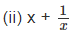(iii) √y – 8

(iv) z5 – ∛z + 8

Sol.

(i) 3x2 – 5x = 3x2 – 5x1 Is a polynomial in one variable.= x1 + x–1 Is not a polynomial as the second term 1/x has degree (–1).

(iii) √y – 8 = y1/2 – 8 Is not a polynomial since, the power of the first term (√y) is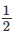, which is not a whole number.

(iv) z5 – ∛z + 8 = z5 – z1/3 + 8
Since, the exponent of the second term is 1/3, which in not a whole number. Therefore, the given expression
is not a polynomial.

Ex. Find the degree of the polynomial :

(i) 5x2 – 6x3 + 8x7 + 6x2

(ii) 2y12 + 3y10 – y15 + y + 3

(iii) x

(iv) 8

Sol. (i) Since the term with highest exponent (power) is 8x7 and its power is 7.

∴ The degree of given polynomial is 7.

(ii) The highest power of the variable is 15. ⇒ degree = 15

(iii) x = x1 ⇒ degree is 1.

(iv) 8 = 8x0 ⇒ degree is 0.

Ex. Write the coefficient of x2 in each of the following :

(i) 2 + x2 + x

(ii) 5 – x2 + x3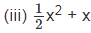(iv) √5x – 1

Sol. (i) In the polynomial 2 + x2 + x the coefficient of x2 is 1.

(ii) In the polynomial 5 – x2 + x3 the coefficient of x2 is -1.

(iii) In the polynomialx2 + x the coefficient of x2 is.

(iv) In the polynomial √5x – 1 the coefficient of x2 = 0.

[We may write √5x – 1 = ax2 + √5x – 1. So the coefficient of x2 is 0]

GEOMETRICAL MEANING OF THE ZEROS OF A POLYNOMIAL

Geometrically the zeros of a polynomials f(x) are the x-co-ordinates of the points where the graph y = f(x) intersects x-axis. To understand it, we will see the geometrical representations of linear and quadratic polynomials.

GEOMETRICAL REPRESENTATION OF THE ZERO OF A LINEAR POLYNOMIAL

Consider a linear polynomial, y = 2x – 5.
The following table lists the values of y corresponding to different values of x.

 x 1 4 y -3 3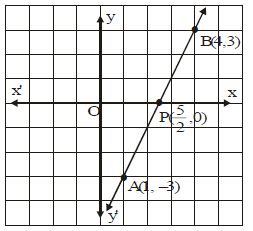On plotting the points A(1, –3) and B(4, 3) and joining them, a straight line is obtained. From, graph we observe that the graph of y = 2x – 5 intersects the x-axis at (5/2,0) whose x-coordinate is 5/2. Also, zero of 2x-5 is 5/2.

Therefore, we conclude that the linear polynomial ax + b has one and only one zero, which is the x-coordinate of the point where the graph of y = ax + b intersects the x-axis

COMPETITION WINDOW

RELATIONSHIP BETWEEN THE ZEROS AND COEFFICIENTS OF A POLYNOMIAL
For a linear polynomial ax + b,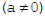, we have,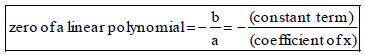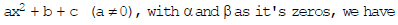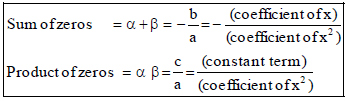If α and β are the zeros of a quadratic polynomial f(x). Then polynomial f(x) is given by

f(x) = K{x2 – (α + β)x + αβ}

or f(x) = K{x2 – (sum of the zeros) x + product of the zeros}

where K is a constant.

Ex. Find the zeroes of the quadratic polynomial 6x2 – 13x + 6 and verify the relation between the zeroes and its coefficients.

Sol. We have,6x2 – 13x + 6 = 6x2 – 4x – 9x + 6 = 2x(3x – 2) – 3(3x – 2) = (3x – 2) (2x – 3)
So, the value of 6x2 – 13x + 6 is 0, when (3x – 2) = 0 or (2x – 3) = 0 i.e.,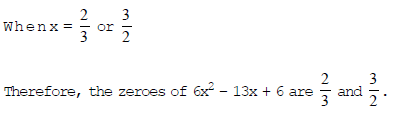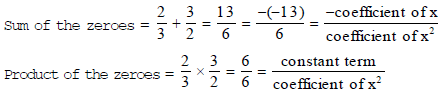Ex. Find the zeroes of the quadratic polynomial 4x2 – 9 and verify the relation between the zeroes and its coefficients.
Sol.
We have,4x2 – 9 = (2x)2 – (3)3 = (2x – 3) (2x + 3)
So, the value of 4x2 – 9 is 0, when 2x – 3 = 0 or 2x + 3 = 0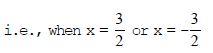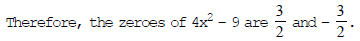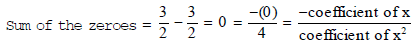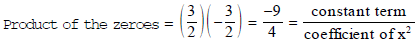REMAINDER THEOREM

If p(x) is any polynomial of degree greater than or equal to 1 and let a be any real number. When p(x) is divided by x– a, then the remainder is equal to p(a).
By division algorithm, we know that Dividend = Divisor × quotient + Remainder

d(x) = p(x) q(x) + r(x)

 REMARK 1.  If a polynomial p(x) is divided by (x + a), the remainder is equal to the value of p(x) at x = – a i.e., p(–a)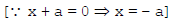2.  If a polynomial p(x) is divided by (ax – b), the remainder is equal to the value of p(x) at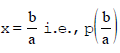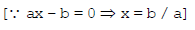3. If a polynomial p(x) is divided by (b – ax), the remainder is equal to the value of p(x) at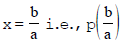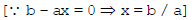Ex. Find the remainder when 4x3 – 3x2 + 2x – 4 is divided by :
(a) x – 1

(b) x + 2

(c) x +Sol. Let p(x) = 4x3 – 3x2 + 2x – 4

(a) When p(x) is divided by (x – 1), then by remainder theorem, the required remainder will be p(1)

p(1) = 4(1)3 – 3(1)2 + 2(1) – 4 = 4 × 1 – 3 × 1 + 2 × 1 – 4 = 4 – 3 + 2 – 4 = – 1

(b) When p(x) is divided by (x + 2) then by remainder theorem, the required remainder will be p(–2).

p(–2) = 4(–2)3 – 3(–2)2 + 2(–2) – 4 = 4 × (–8) – 3 × 4 – 4 – 4 = – 32 – 12 – 8 = – 52

(c) When p(x) is divided by,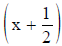then by remainder theorem, the required remainder will be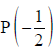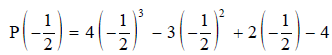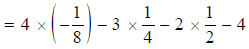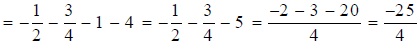Ex. Find the remainder when the polynomial f(x) = 2x4 – 6x3 + 2x2 – x + 2 is divided by x + 2.

Sol. when f(x) is divided by (x + 2), then by remainder theorem remainder will be f(–2).

Now, f(x) = 2x4 – 6x3 + 2x2 – x + 2

⇒ f(–2) = 2(–2)4 – 6(–2)3 + 2(–2)2 – (–2) + 2

⇒ f(–2) = 2 × 16 – 6 × (–8) + 2 × 4 + 2 + 2

⇒ f(–2) = 32 + 48 + 8 + 2 + 2 = 92

Ex. If the polynomials ax3 + 4x2 + 3x – 4 and x3 – 4x + a leave the same remainder when divided by (x – 3), find the value of a.

Sol. Let p(x) = ax3 + 4x2 + 3x – 4 and q(x) = x3 – 4x + a be the given polynomials. The remainders when p(x) and q(x) are divided by (x – 3) are p(3) and q(3) respectively.

By the given condition, we have p(3) = q(3)

⇒ a × (3)3 + 4 × (3)2 + 3 × 3 – 4 = (3)3 – 4 × 3 + a

⇒ 27a + 36 + 9 – 4 = 27 – 12 + a

⇒ 26a + 26 = 0

⇒ 26a = – 26 ⇒ a = – 1

The document Zeroes of a Polynomial and Remainder Theorem - Polynomials, Class 9, Mathematics - Notes - Class 9 is a part of Class 9 category.
All you need of Class 9 at this link: Class 9
 Use Code STAYHOME200 and get INR 200 additional OFF

Track your progress, build streaks, highlight & save important lessons and more!

,

,

,

,

,

,

,

,

,

,

,

,

,

,

,

,

,

,

,

,

,

,

,

,

,

,

,

;